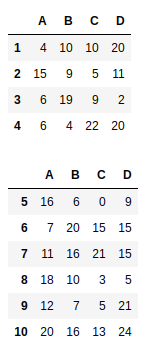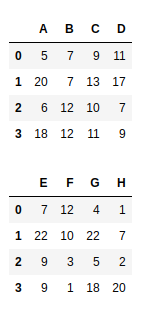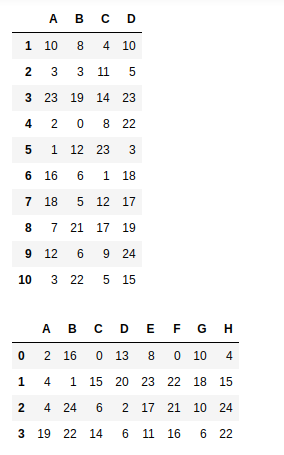# How To Concatenate Two or More Pandas DataFrames?

• Difficulty Level : Basic
• Last Updated : 05 Jun, 2021

Let’s understand how we can concatenate two or more Data Frames. A concatenation of two or more data frames can be done using pandas.concat() method. concat() in pandas works by combining Data Frames across rows or columns. We can concat two or more data frames either along rows  (axis=0) or along columns (axis=1)

Step 1: Import numpy and pandas libraries.

Attention geek! Strengthen your foundations with the Python Programming Foundation Course and learn the basics.

To begin with, your interview preparations Enhance your Data Structures concepts with the Python DS Course. And to begin with your Machine Learning Journey, join the Machine Learning - Basic Level Course

## Python3

 `import` `pandas as pd``import` `numpy as np`

Step 2: Create two Data Frames which we will be concatenating now. For creating Data frames we will be using numpy and pandas.

## Python3

 `df1 ``=` `pd.DataFrame(np.random.randint(``25``, size``=``(``4``, ``4``)),``                   ``index``=``[``"1"``, ``"2"``, ``"3"``, ``"4"``],``                   ``columns``=``[``"A"``, ``"B"``, ``"C"``, ``"D"``])` `df2 ``=` `pd.DataFrame(np.random.randint(``25``, size``=``(``6``, ``4``)),``                   ``index``=``[``"5"``, ``"6"``, ``"7"``, ``"8"``, ``"9"``, ``"10"``],``                   ``columns``=``[``"A"``, ``"B"``, ``"C"``, ``"D"``])` `df3 ``=` `pd.DataFrame(np.random.randint(``25``, size``=``(``4``, ``4``)),``                   ``columns``=``[``"A"``, ``"B"``, ``"C"``, ``"D"``])` `df4 ``=` `pd.DataFrame(np.random.randint(``25``, size``=``(``4``, ``4``)),``                   ``columns``=``[``"E"``, ``"F"``, ``"G"``, ``"H"``])` `display(df1, df2, df3, df4)`

Output:Step 3: Now we need to pass the two data frames to the contact() method in the form of a list and mention in which axis you want to concat.

## Python3

 `# concatenating df1 and df2 along rows``vertical_concat ``=` `pd.concat([df1, df2], axis``=``0``)` `# concatenating df3 and df4 along columns``horizontal_concat ``=` `pd.concat([df3, df4], axis``=``1``)` `display(vertical_concat, horizontal_concat)`

Output:My Personal Notes arrow_drop_up